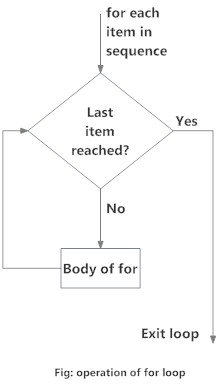# Python for Loop

In this article, you'll learn to iterate over a sequence of elements using the different variations of for loop.

## What is for loop in Python?

The for loop in Python is used to iterate over a sequence (list, tuple, string) or other iterable objects. Iterating over a sequence is called traversal.

### Syntax of for Loop

```for val in sequence:
Body of for
```

Here, `val` is the variable that takes the value of the item inside the sequence on each iteration.

Loop continues until we reach the last item in the sequence. The body of for loop is separated from the rest of the code using indentation.

### Flowchart of for Loop### Example: Python for Loop

```# Program to find the sum of all numbers stored in a list

# List of numbers
numbers = [6, 5, 3, 8, 4, 2, 5, 4, 11]

# variable to store the sum
sum = 0

# iterate over the list
for val in numbers:
sum = sum+val

# Output: The sum is 48
print("The sum is", sum)
```

when you run the program, the output will be:

```The sum is 48
```

## The range() function

We can generate a sequence of numbers using `range()` function. `range(10)` will generate numbers from 0 to 9 (10 numbers).

We can also define the start, stop and step size as `range(start,stop,step size)`. step size defaults to 1 if not provided.

This function does not store all the values in memory, it would be inefficient. So it remembers the start, stop, step size and generates the next number on the go.

To force this function to output all the items, we can use the function `list()`.

The following example will clarify this.

```# Output: range(0, 10)
print(range(10))

# Output: [0, 1, 2, 3, 4, 5, 6, 7, 8, 9]
print(list(range(10)))

# Output: [2, 3, 4, 5, 6, 7]
print(list(range(2, 8)))

# Output: [2, 5, 8, 11, 14, 17]
print(list(range(2, 20, 3)))	```

We can use the range() function in for loops to iterate through a sequence of numbers. It can be combined with the `len()` function to iterate though a sequence using indexing. Here is an example.

```# Program to iterate through a list using indexing

genre = ['pop', 'rock', 'jazz']

# iterate over the list using index
for i in range(len(genre)):
print("I like", genre[i])
```

When you run the program, the output will be:

```I like pop
I like rock
​I like jazz```

## for loop with else

A for loop can have an optional else block as well. The `else` part is executed if the items in the sequence used in for loop exhausts.

break statement can be used to stop a for loop. In such case, the else part is ignored.

Hence, a for loop's else part runs if no break occurs.

Here is an example to illustrate this.

```digits = [0, 1, 5]

for i in digits:
print(i)
else:
print("No items left.")```

When you run the program, the output will be:

```0
1
5
No items left.```

Here, the for loop prints items of the list until the loop exhausts. When the for loop exhausts, it executes the block of code in the `else` and prints

`No items left.`